Algebra 2 6-4 Complete Lesson: Rational Exponents
starstarstarstarstarstarstarstarstarstar
4.5 (1 rating)
by Matthew Richardson
| 37 Questions
Note from the author:
A complete formative lesson with embedded slideshow, mini lecture screencasts, checks for understanding, practice items, mixed review, and reflection. I create these assignments to supplement each lesson of Pearson's Common Core Edition Algebra 1, Algebra 2, and Geometry courses. See also mathquest.net and twitter.com/mathquestEDU.The outlined content above was added from outside of Formative.1
2
1
10 pts
Solve It! On the canvas, show how to cut the three linked 1-squares into congruent pieces, each with size ¾.
2
10 pts
Solve It! Reasoning: Explain why you cannot cut them into congruent pieces, each with size 4/5.
You cannot cut the three 1-squares into congruent 4/5-pieces because 3 ÷ 4/5 is not a whole number.
You cannot cut the three 1-squares into congruent 4/5-pieces because 3 × 4/5 is not a whole number.
You cannot cut the three 1-squares into congruent 4/5-pieces because 3 is an odd number.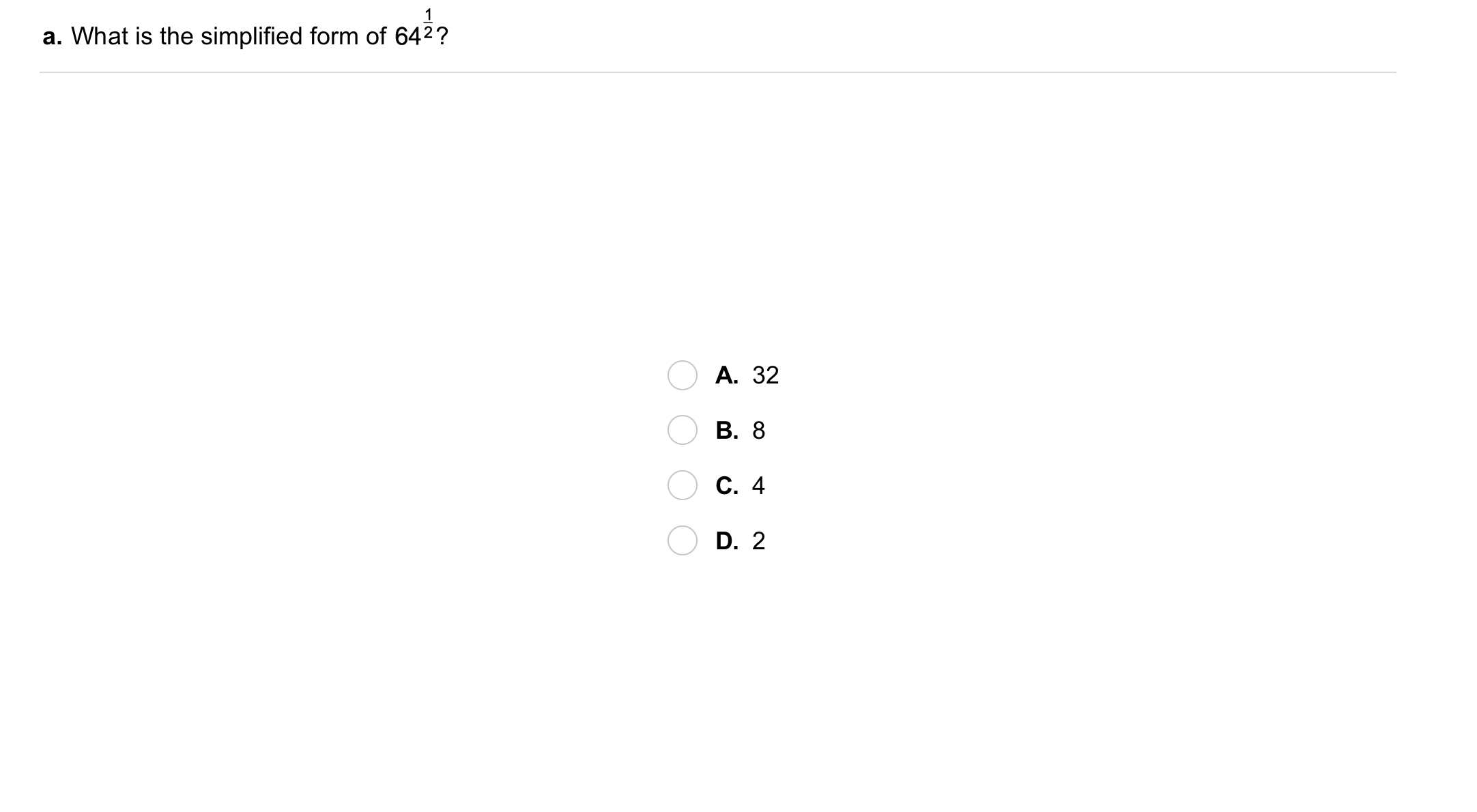3
3
10 pts
Problem 1 Got It?
A
B
C
D4
4
10 pts
Problem 1 Got It?
A
B
C
D5
5
10 pts
Problem 1 Got It?
A
B
C
D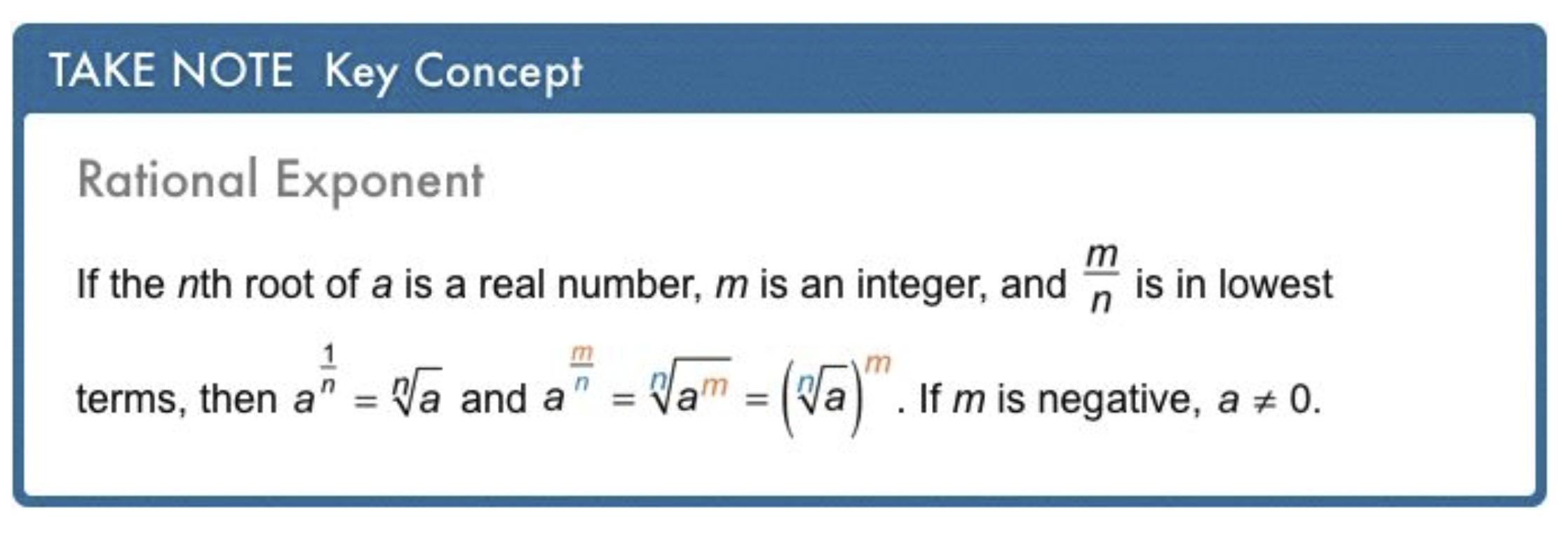6
10 pts
Problem 2 Got It? What is the expression in radical form?

7
10 pts
Problem 2 Got It? What is the expression in radical form?

8
10 pts
Problem 2 Got It? What is the expression in exponential form?

9
10 pts
Problem 2 Got It? What is the expression in exponential form?

10
10 pts
Problem 2 Got It? Reasoning: Refer to the definition of rational exponent. Explain the need for the restriction that a ≠ 0 if m is negative.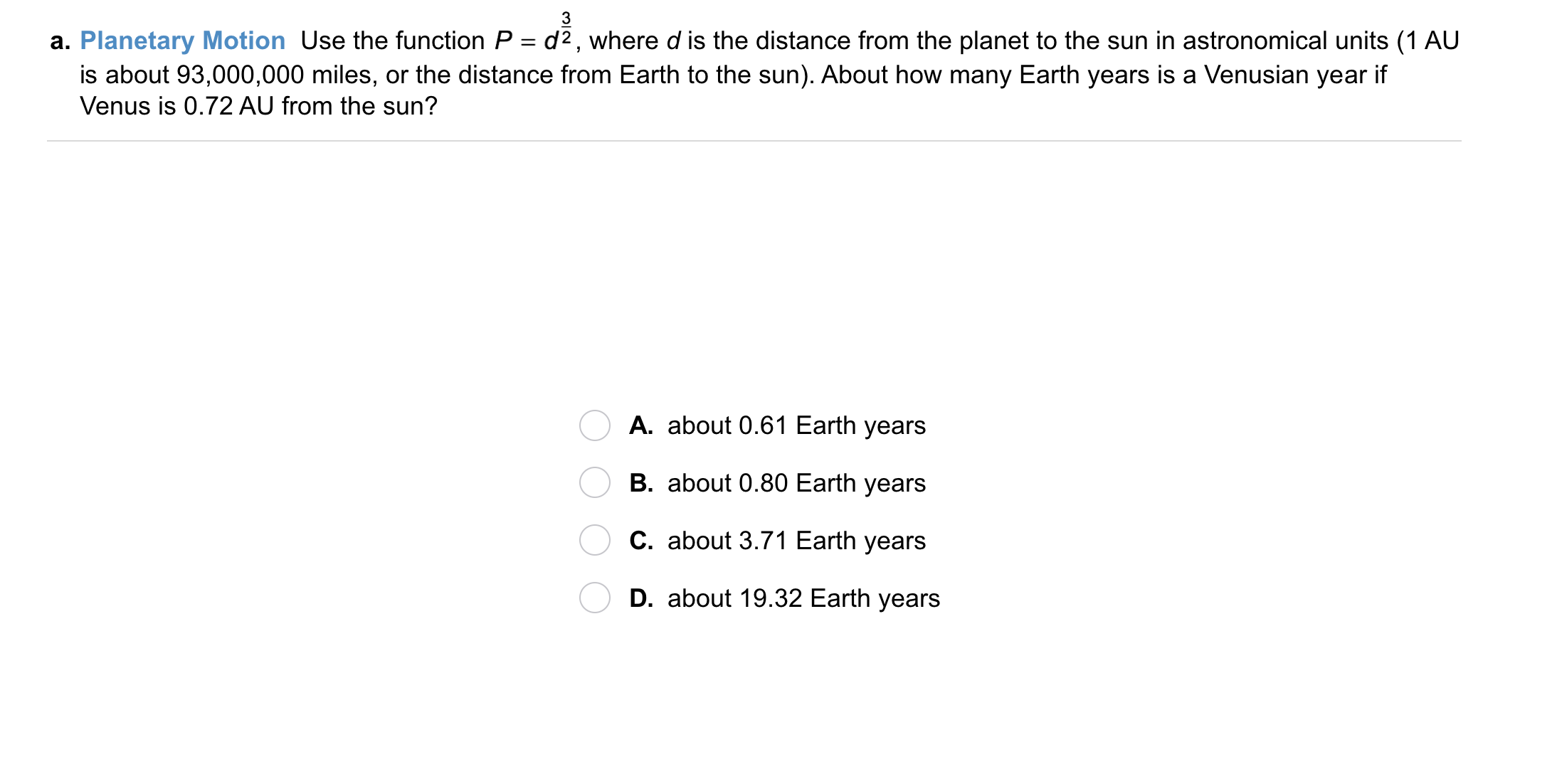11
11
10 pts
Problem 3 Got It? Planetary Motion: Use the function
where d is the distance from the planet to the sun in astronomical units (1 AU is about 93,000,000 miles, or the distance from Earth to the sun). About how many Earth years is a Venusian year if Venus is 0.72 AU from the sun?
A
B
C
D12
12
10 pts
Problem 3 Got It? Planetary Motion: Use the function
where d is the distance from the planet to the sun in astronomical units (1 AU is about 93,000,000 miles, or the distance from Earth to the sun). About how many Earth years is a Jovian year if Jupiter is 5.46 AU from the sun?
A
B
C
D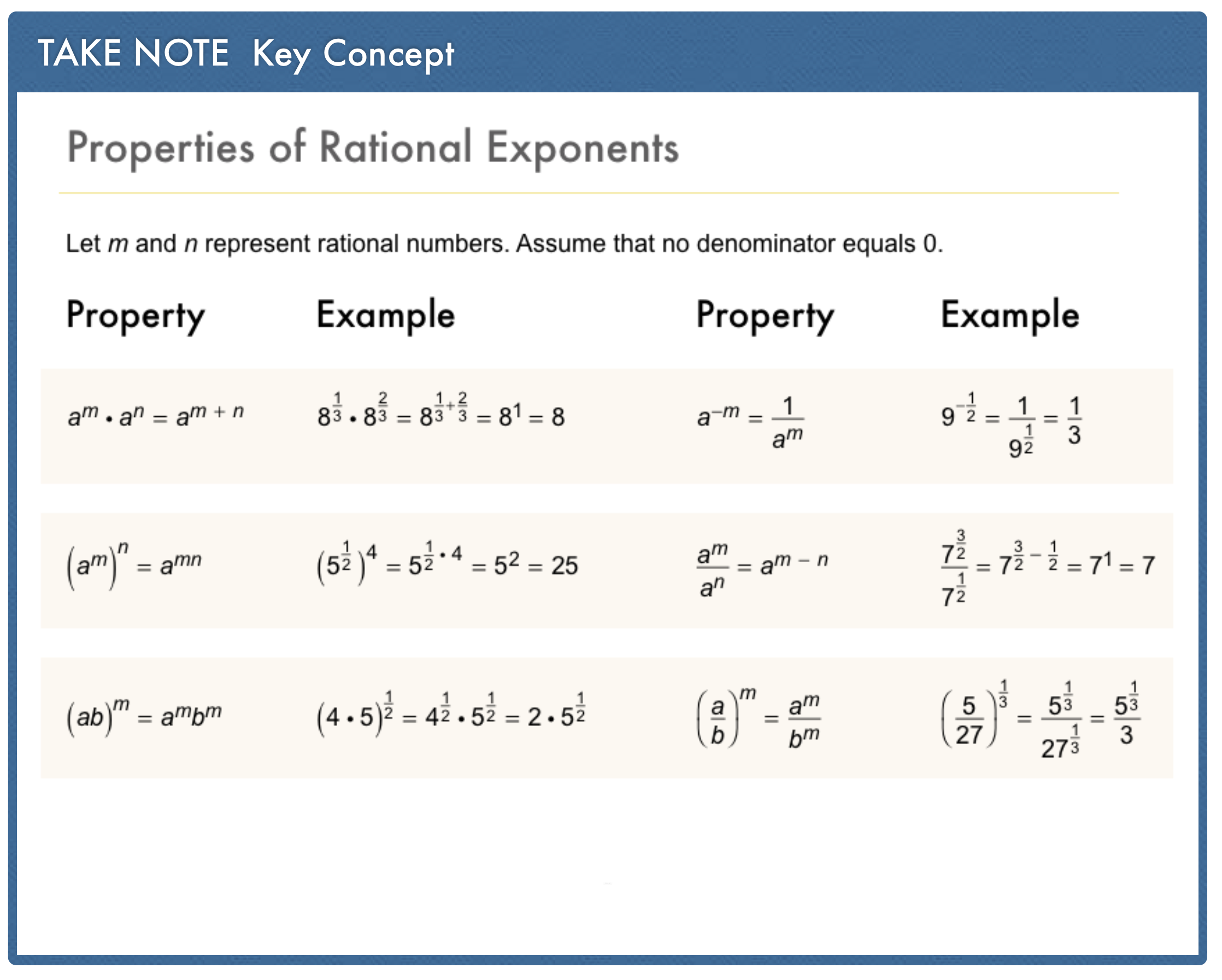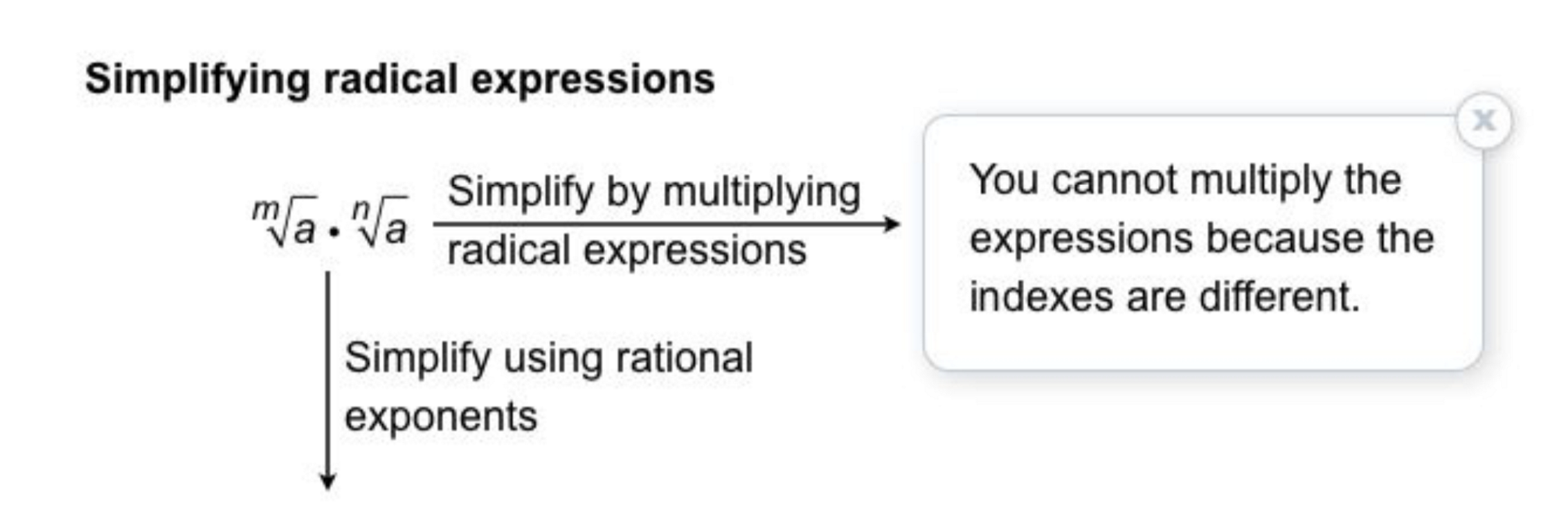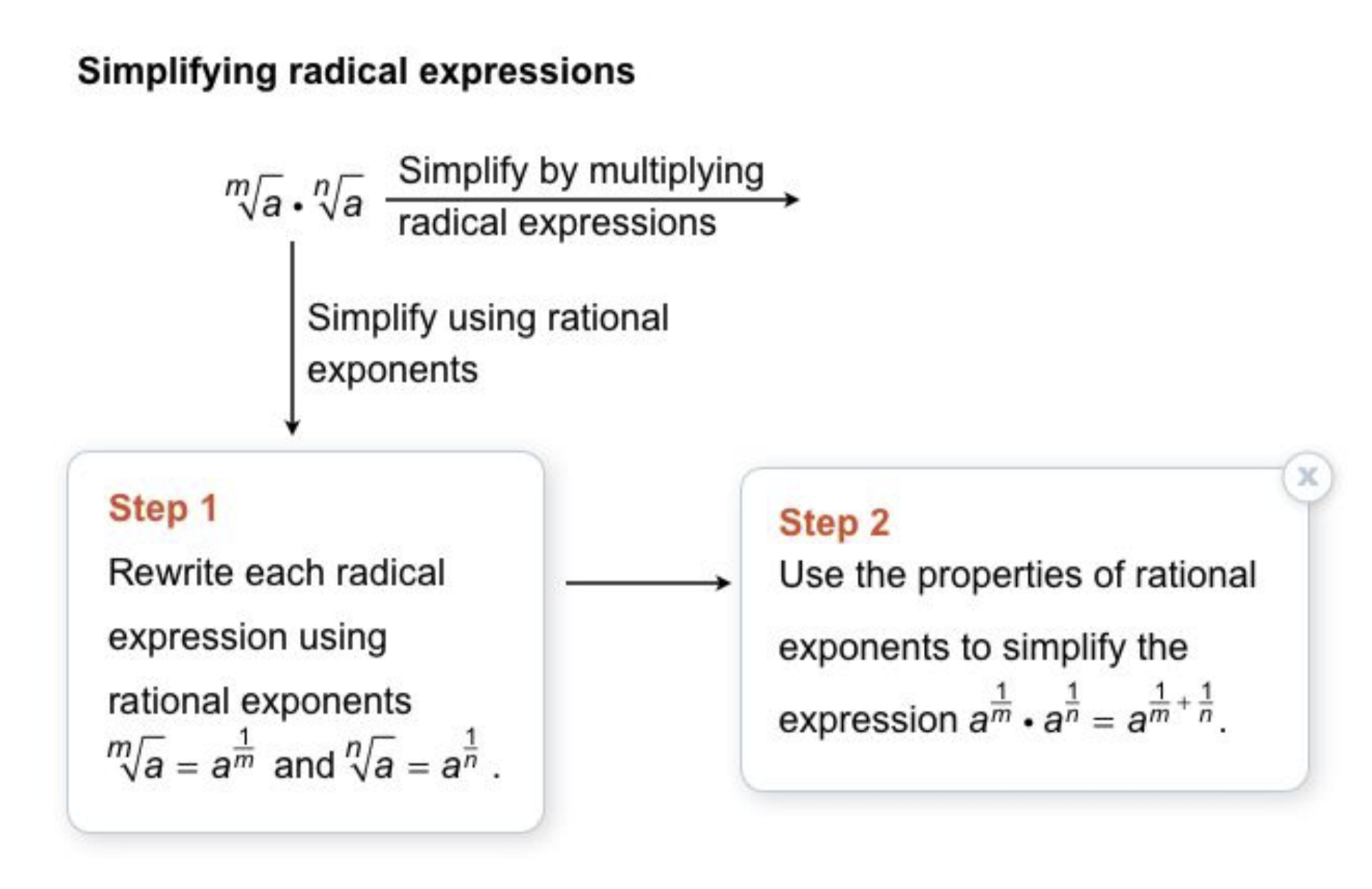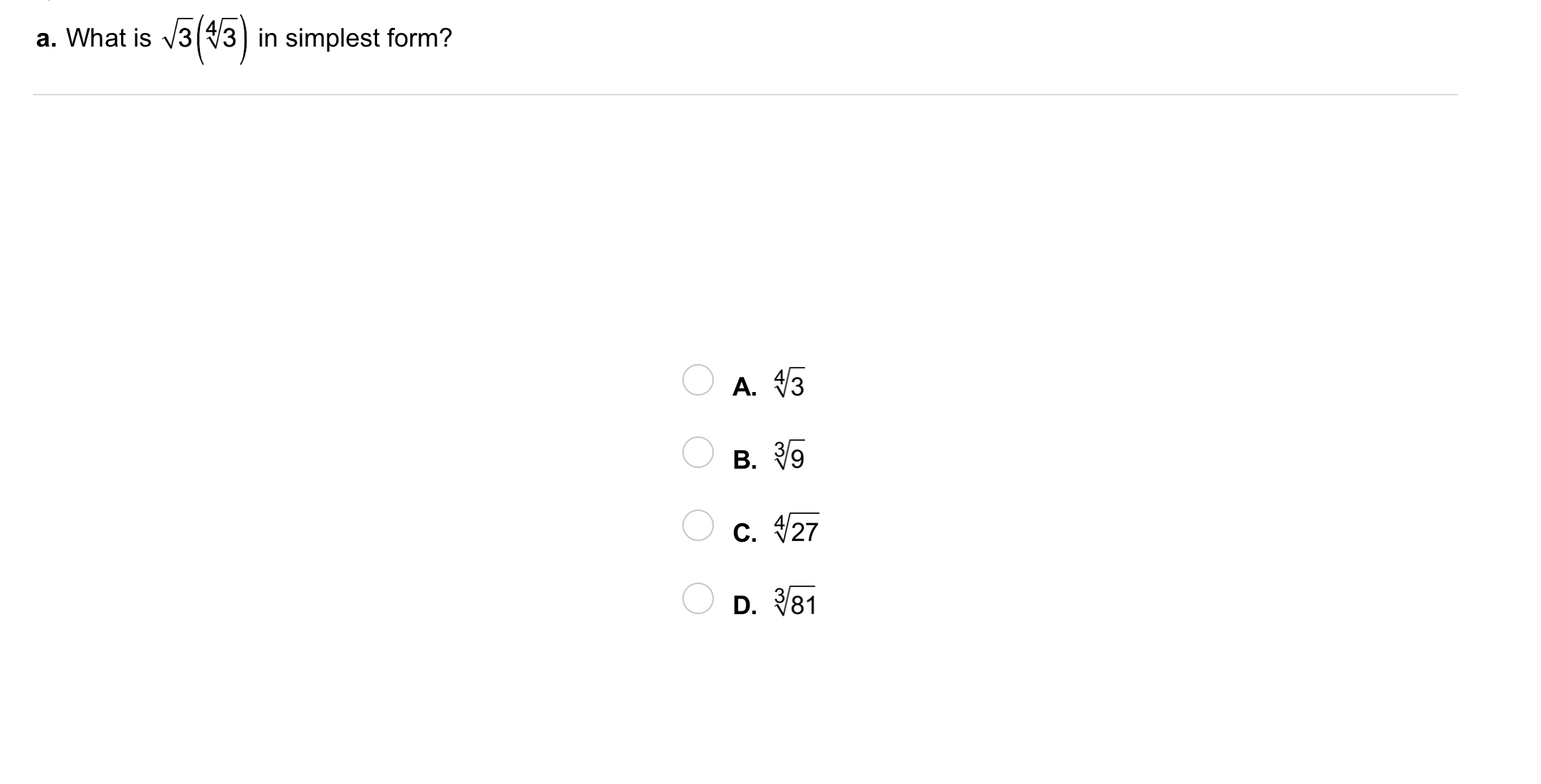13
13
10 pts
Problem 4 Got It?
A
B
C
D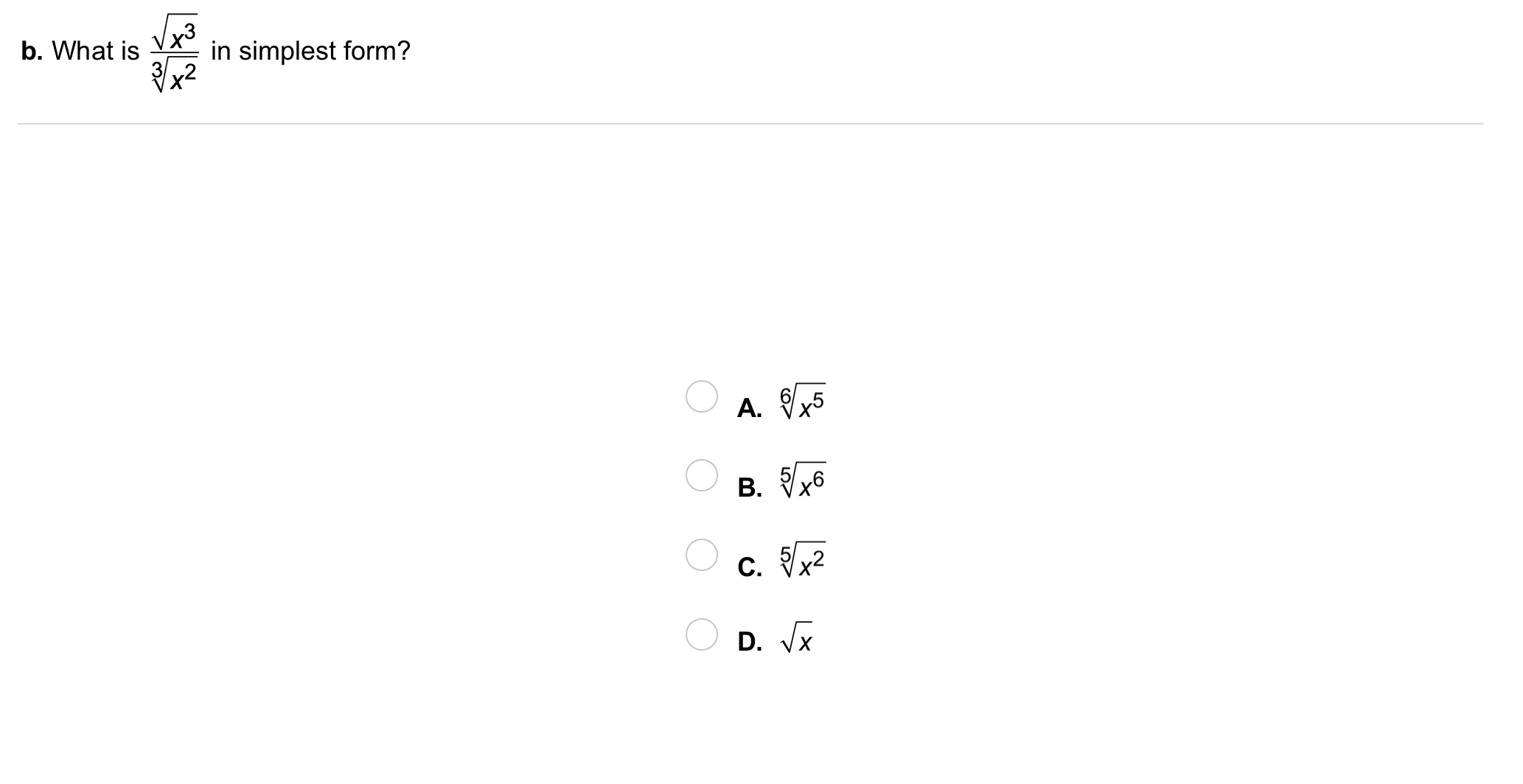14
14
10 pts
Problem 4 Got It?
A
B
C
D15
15
10 pts
Problem 4 Got It?
A
B
C
D16
16
10 pts
Problem 5 Got It?
A
B
C
D17
17
10 pts
Problem 5 Got It?
A
B
C
D18
18
10 pts
Problem 5 Got It?
A
B
C
D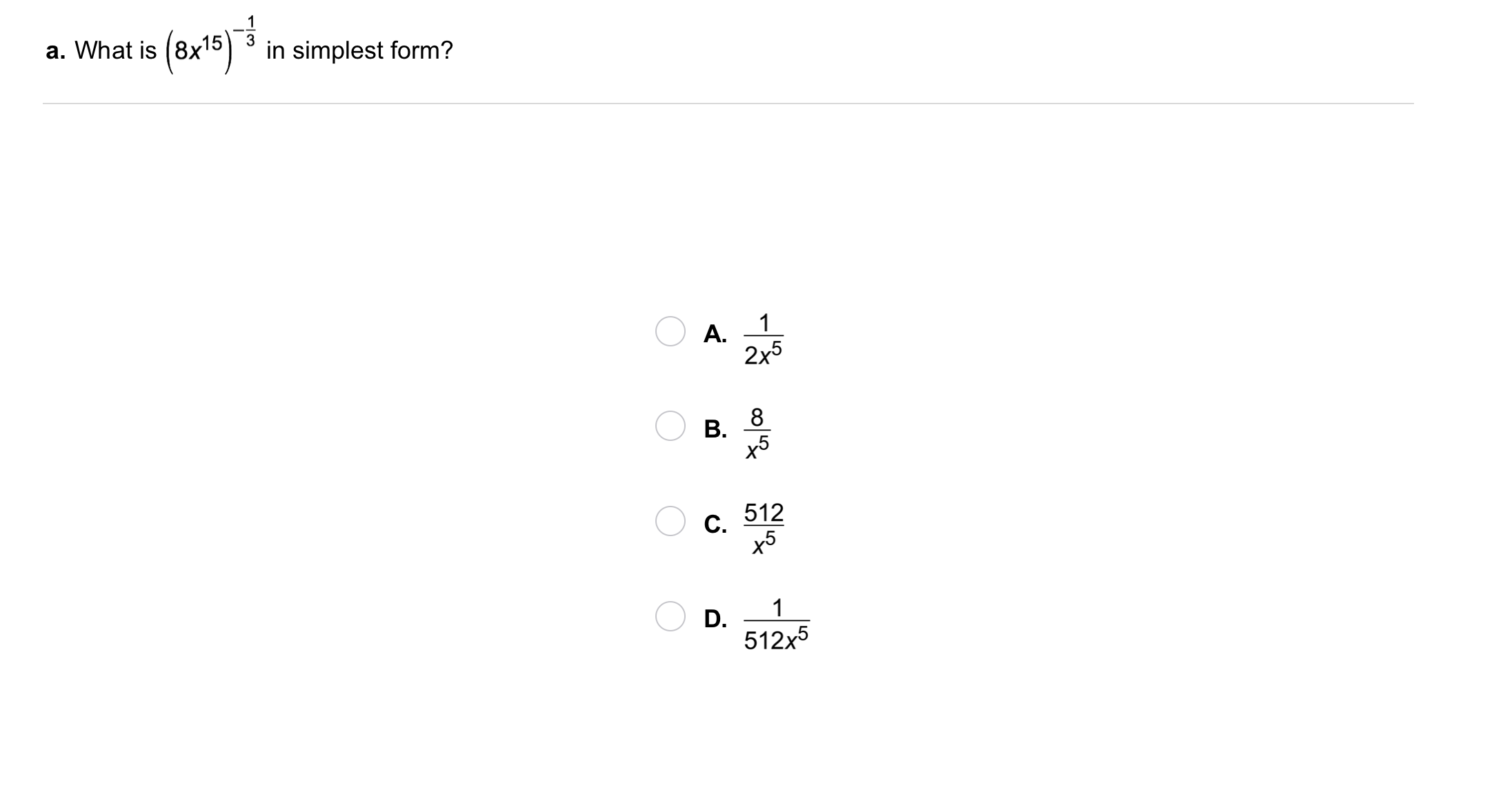19
19
10 pts
Problem 6 Got It?
A
B
C
D20
20
10 pts
Problem 6 Got It?
A
B
C
D21
21
10 pts
A
B
C
D22
22
10 pts
A
B
C
D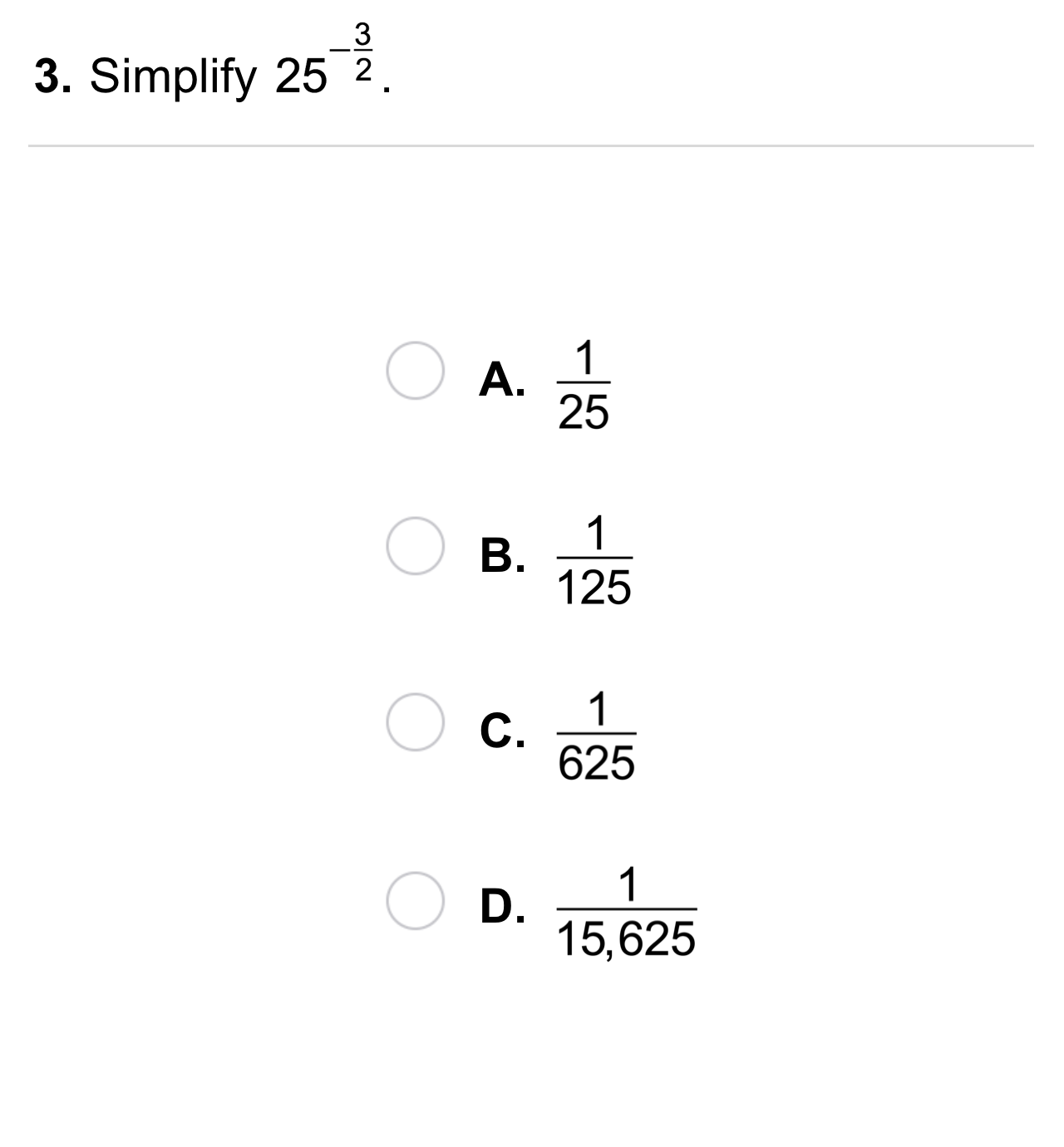23
23
10 pts
A
B
C
D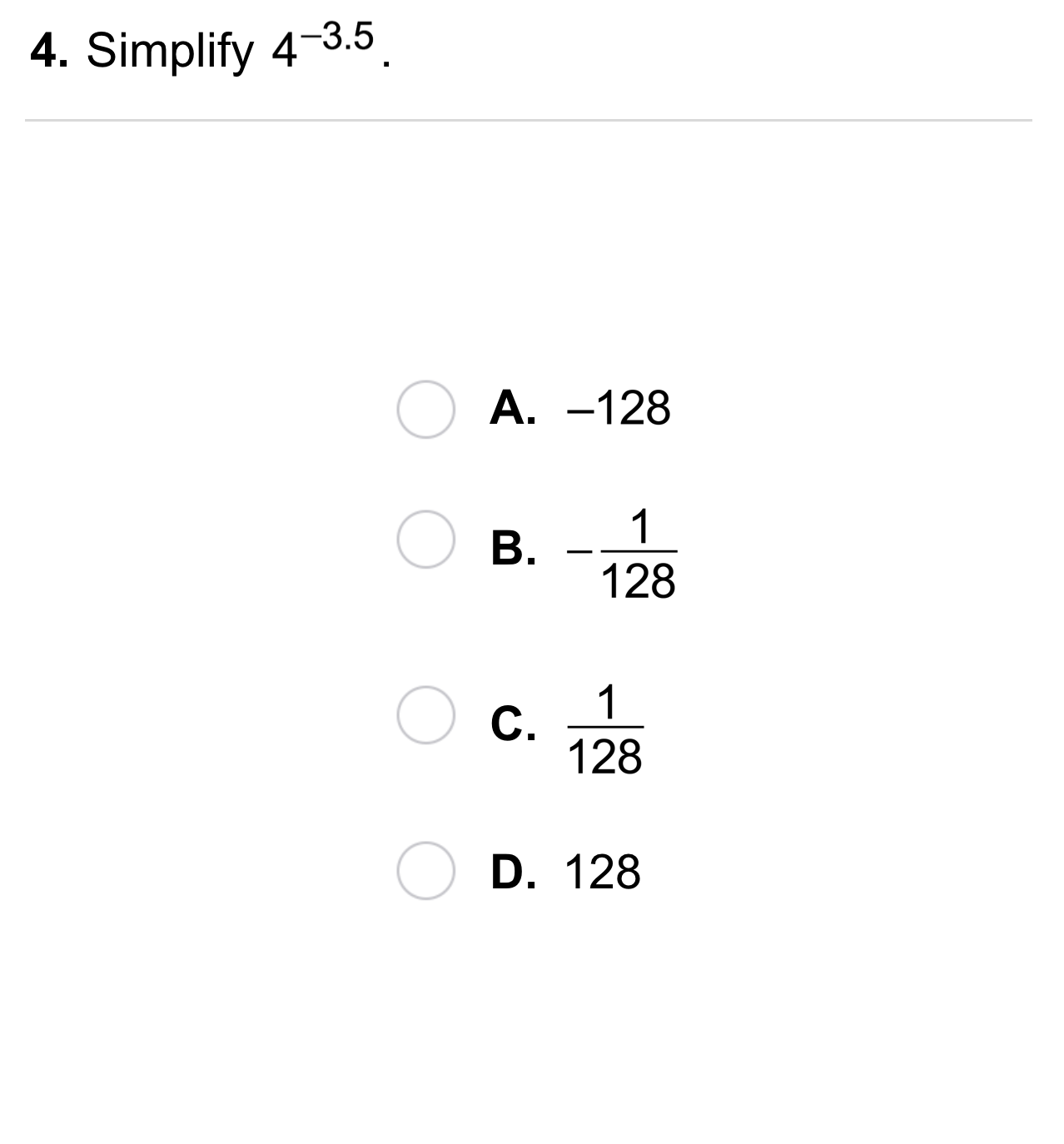24
24
10 pts
A
B
C
D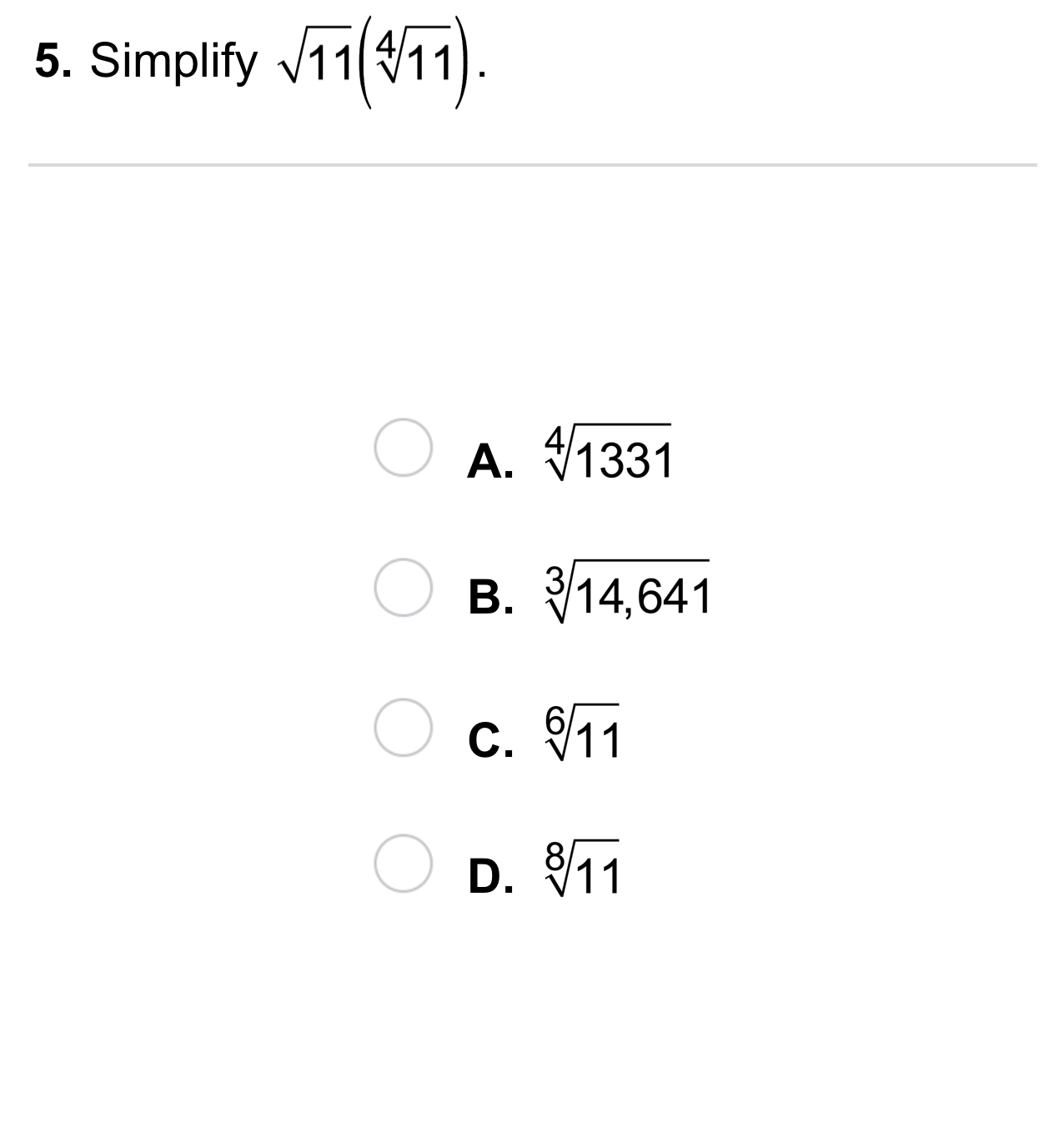25
25
10 pts
A
B
C
D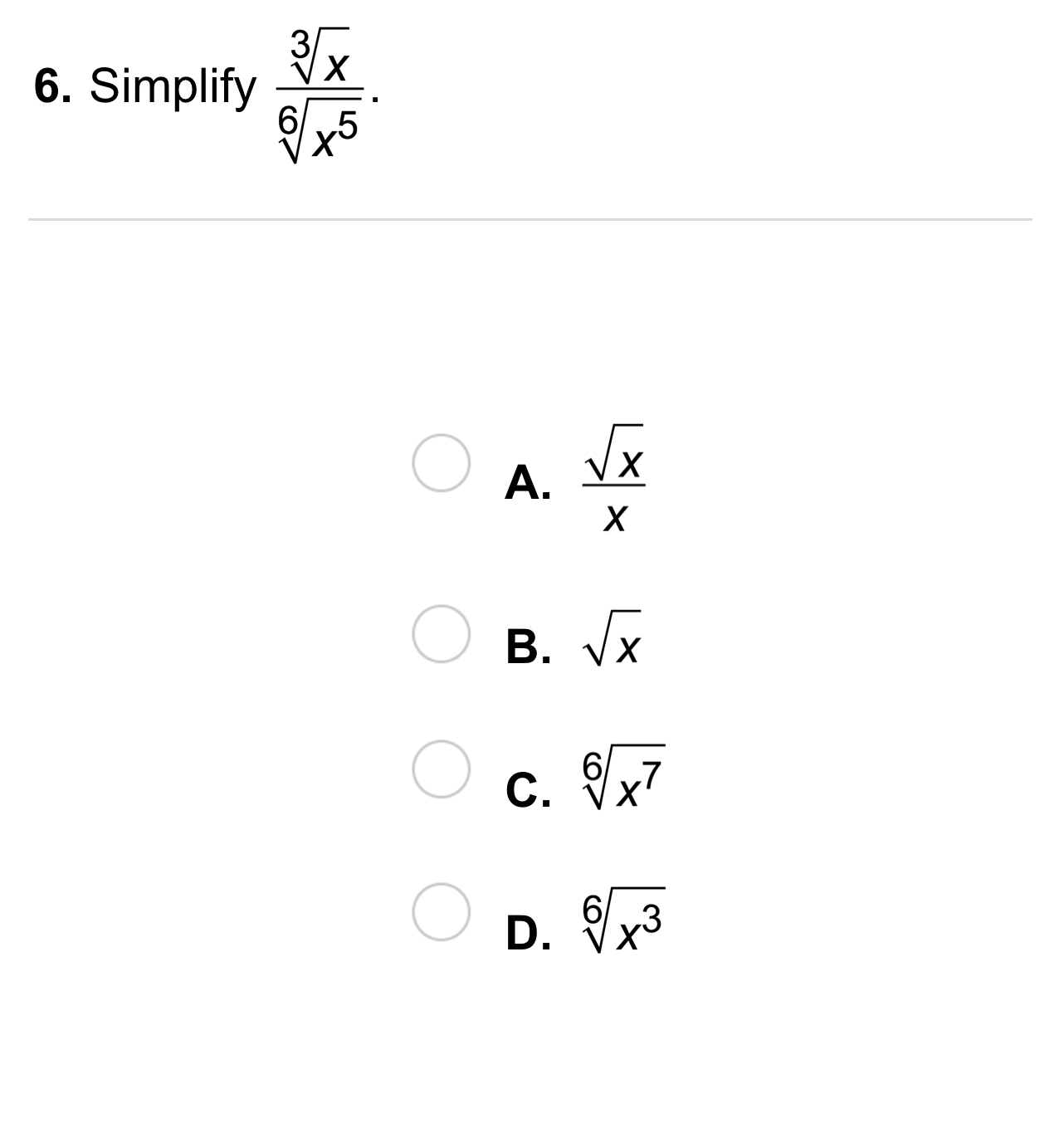26
26
10 pts
A
B
C
D27
27
10 pts
Error Analysis: Explain why this simplification is incorrect.29
10 pts
Review Lesson 6-3: Match each radical expression on the left with its simplified form on the right.

30
10 pts
Review Lesson 4-4: Factor the expression completely.

31
10 pts
Review Lesson 4-4: Factor the expression completely.

32
10 pts
Review Lesson 4-4: Factor the expression completely.

33
10 pts
Review Lesson 4-5: Solve by factoring. Match the solutions on the left with the equation(s) they solve on the right.
• 1
• -1/3
• -3/2
• -3
• 234
50 pts
Vocabulary [Real Numbers] Review: Use the terms in the box to complete the diagram. Then give an example of each type of number.35
10 pts
Use Your Vocabulary: Complete each sentence with the correct form of the word convert.
• convert
• converted
• convertible
• conversion
• NOUN: Travelers to Europe calculate the __?__ of dollars to euros.
• ADJECTIVE: A __?__ sofa is a couch by day and a bed by night.
• VERB: To __?__ fractions to decimals, divide the numerator by the denominator.
36
100 pts
Notes: Take a clear picture or screenshot of your Cornell notes for this lesson. Upload it to the canvas. Zoom and pan as needed.

For a refresher on the Cornell note-taking system, click here.
37
10 pts
Reflection: Math Success
Add to my formatives list

Formative uses cookies to allow us to better understand how the site is used. By continuing to use this site, you consent to the Terms of Service and Privacy Policy.